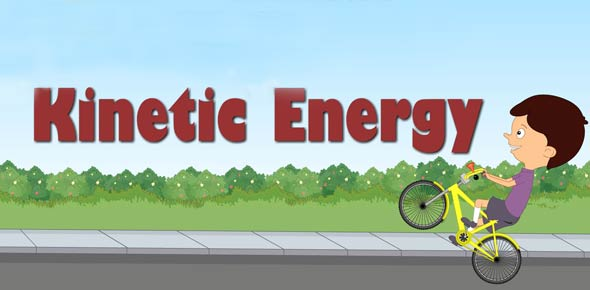# Quiz 7: Rotational Kinetic Energy

10 Questions | Total Attempts: 288Settings• 1.
When playing billiards, the collisions between the balls are best described as __________________.
• A.

Elastic

• B.

Inelastic

• C.

Expensive

• D.

Exhaustive

• 2.
If two objects collide with each other, _______________.
• A.

The sum of their momentums after the collision is equal to the sum of their momentums before the collision

• B.

The momentum before the collision is always greater than the total momentum after the collision

• C.

The object with greater mass always has greater momentum

• D.

They both lose momentum at the moment of impact

• 3.
Momentum is the product of ___________.
• A.

Mass times velocity

• B.

Force times distance

• C.

Speed times acceleration

• D.

Time and space

• 4.
The kinetic energy of an object will increase the most if you __________________.
• A.

Double the velocity of the object

• B.

Double the mass of the object

• C.

Double the potential energy

• D.

Double the temperature of the object

• 5.
A person jumps to the ground from a platform 10 feet high.  Which of the following is true?
• A.

The person has more kinetic energy before they jump than after they jump.

• B.

The person has less potential energy at the top of the platform than in the air.

• C.

The person's potential energy at the instant they jump is approximately equal to their kinetic energy the instant they land.

• D.

The person's kinetic energy at the instant they jump is approximately double their potential energy the instant they land.

• 6.
You have 3 round objects of equal mass and equal radius, but different shapes.  Which of the following shapes will have the largest moment of inertia?
• A.

A hollow ring

• B.

A solid disk

• C.

A solid sphere

• D.

They are all equal

• 7.
A round object is at the top of a hill.  Assuming that friction does not slow it down at all, will it go down the hill faster if it just slides down without rotating, or if it rolls down?
• A.

Sliding

• B.

Rolling

• C.

If friction doesn't slow it down, then they are the same

• D.

It depends on whether or not the object is hollow

• 8.
You take an object of irregular shape and draw a big, red dot right on its center of mass.  What path will the red dot trace out if you throw the object spinning through the air?
• A.

A parabola

• B.

A half-circle

• C.

An irregular path since the object is not perfectly round

• D.

You can't tell unless you know how fast the object is spinning

• 9.
A ball rolls down a hill.  The potential energy of the ball at the top of the hill is equal to...
• A.

The translational kinetic energy plus the rotational kinetic energy at the bottom of the hill

• B.

1/2 mv^2 at the bottom of the hill

• C.

It depends on the radius of the ball

• D.

Less than 1/2 mv^2 at the bottom of the hill

• 10.
is the formula for:
• A.

Moment of inertia

• B.

Rotational kinetic energy

• C.

Total kinetic energy of a moving object

• D.

Potential energy of rotation

Related TopicsBack to top